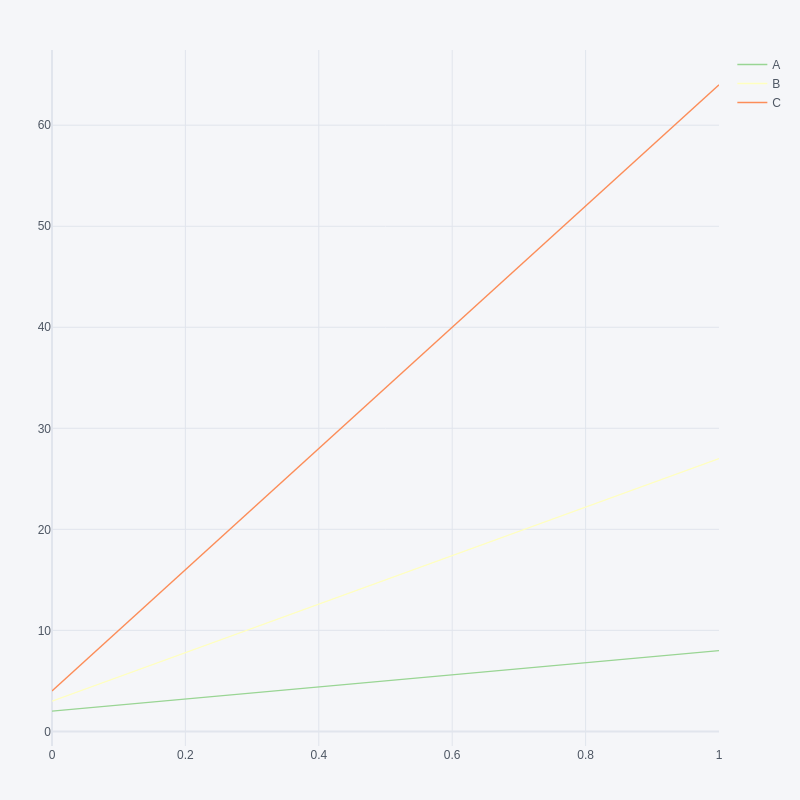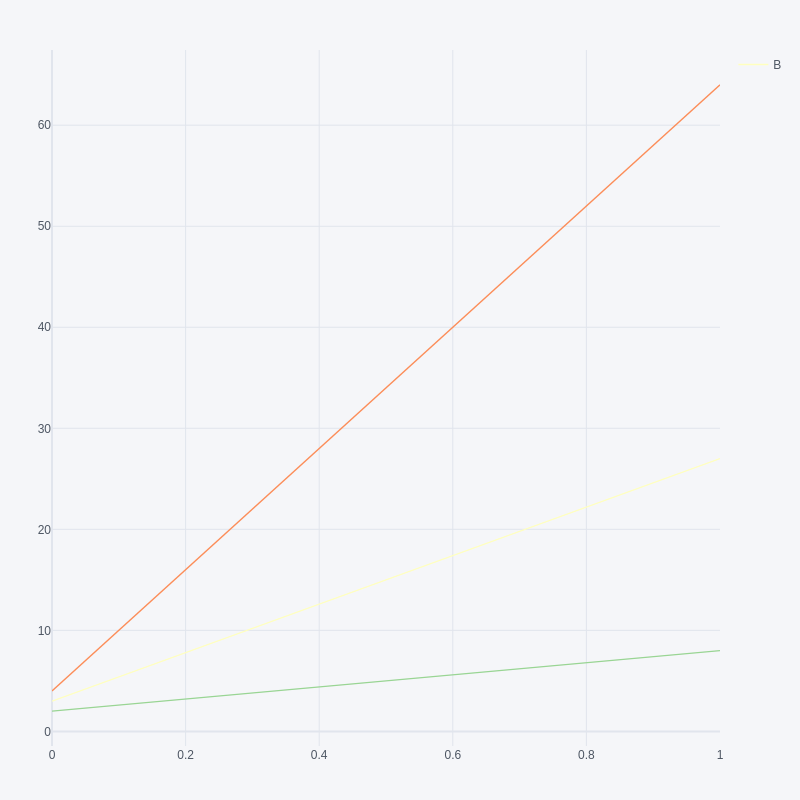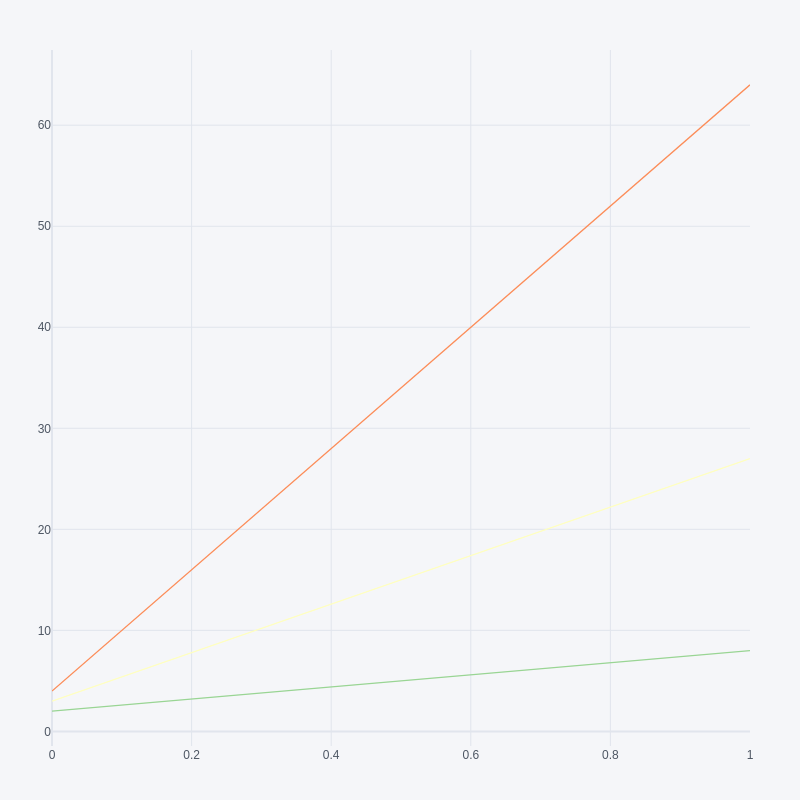Open in App
Not now

# Hide legend entries in a plotly figure in Python

• Last Updated : 22 Nov, 2021

In this article, we are going to see how to hide legend entries in a plotly figure using Python.

The Fig below shows the plot without hiding the legend entries:## Method 1: Setting showlegend property by the name of the trace

Here we are going to set showlegend property to remove the legend entries in a plotly figure.

## Python3

 `import` `pandas as pd ` `import` `plotly.offline as py ` `import` `plotly.graph_objs as go ` `import` `cufflinks as cf ` `cf.go_offline() ` ` `  `df ``=` `pd.DataFrame(data``=``[[``2``, ``3``, ``4``], [``8``, ``27``, ``64``]], ` `                  ``columns``=``[``'A'``, ``'B'``, ``'C'``]) ` ` `  `# get figure property ` `fig ``=` `df.iplot(kind``=``'scatter'``, asFigure``=``True``) ` ` `  `# set showlegend property by name of trace ` `for` `trace ``in` `fig[``'data'``]: ` `    ``if``(trace[``'name'``] !``=` `'B'``): ` `        ``trace[``'showlegend'``] ``=` `False` ` `  `# generate webpage ` `py.plot(fig) `

Output:## Method 2: Using the update function, remove all the entries

Here we are going to use the update function to remove all the entries

## Python3

 `import` `pandas as pd ` `import` `plotly.offline as py ` `import` `plotly.graph_objs as go ` `import` `cufflinks as cf ` `cf.go_offline() ` ` `  `df ``=` `pd.DataFrame(data``=``[[``2``, ``3``, ``4``], [``8``, ``27``, ``64``]],  ` `                  ``columns``=``[``'A'``, ``'B'``, ``'C'``]) ` ` `  `# get figure property ` `fig ``=` `df.iplot(kind``=``'scatter'``, asFigure``=``True``) ` ` `  `# set layout_showlegend=False ` `fig.update(layout_showlegend``=``False``) ` ` `  `# generate webpage ` `py.plot(fig) `

Output:My Personal Notes arrow_drop_up
Related Articles